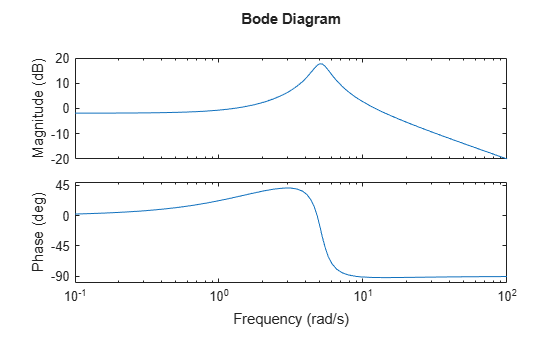# Numeric Values of Frequency-Domain Characteristics of SISO Model

This example shows how to obtain numeric values of several frequency-domain characteristics of a SISO dynamic system model, including the peak gain, dc gain, system bandwidth, and the frequencies at which the system gain crosses a specified frequency.

Create a transfer function model and plot its frequency response.

```H = tf([10,21],[1,1.4,26]); bodeplot(H)```Plotting the frequency response gives a rough idea of the frequency-domain characteristics of the system. `H` includes a pronounced resonant peak, and rolls off at 20 dB/decade at high frequency. It is often desirable to obtain specific numeric values for such characteristics.

Calculate the peak gain and the frequency of the resonance.

```[gpeak,fpeak] = getPeakGain(H); gpeak_dB = mag2db(gpeak)```
```gpeak_dB = 17.7596 ```

`getPeakGain` returns both the peak location `fpeak` and the peak gain `gpeak` in absolute units. Using `mag2db` to convert `gpeak` to decibels shows that the gain peaks at almost 18 dB.

Find the band within which the system gain exceeds 0 dB, or 1 in absolute units.

`wc = getGainCrossover(H,1)`
```wc = 2×1 1.2582 12.1843 ```

`getGainCrossover` returns a vector of frequencies at which the system response crosses the specified gain. The resulting `wc` vector shows that the system gain exceeds 0 dB between about 1.3 and 12.2 rad/s.

Find the dc gain of `H`.

The Bode response plot shows that the gain of `H` tends toward a finite value as the frequency approaches zero. The `dcgain` command finds this value in absolute units.

`k = dcgain(H);`

Find the frequency at which the response of `H` rolls off to –10 dB relative to its dc value.

`fb = bandwidth(H,-10);`

`bandwidth` returns the first frequency at which the system response drops below the dc gain by the specified value in dB.